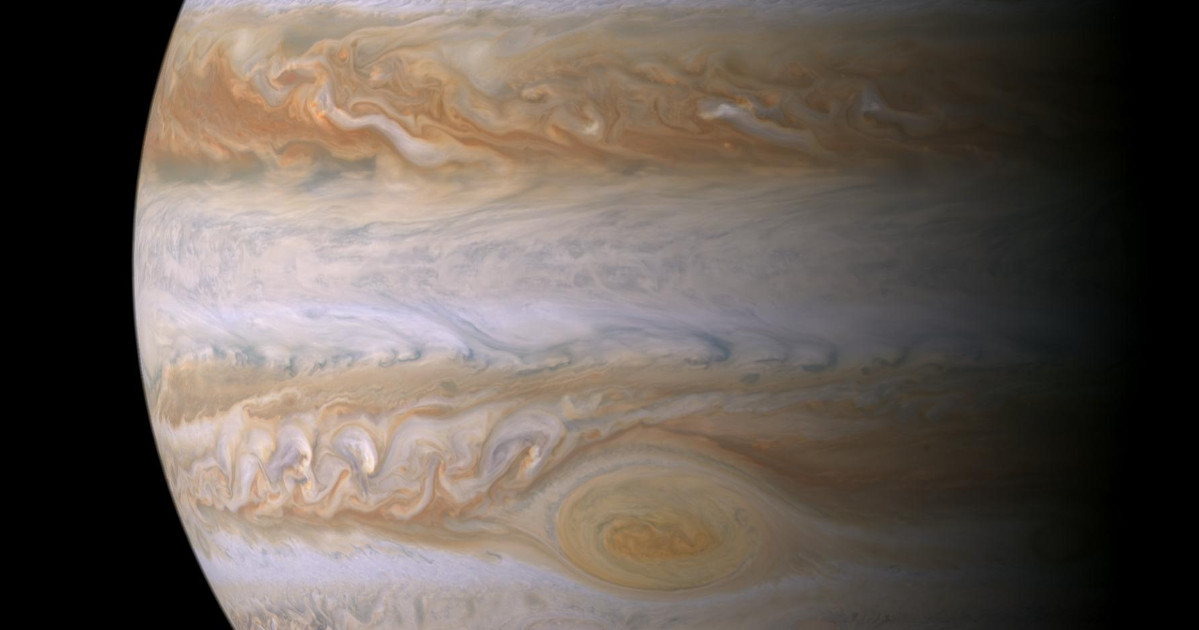# Jupyter Notebook Templates for Data ScienceI love Jupyter notebooks (even if I have strong opinions about their misuse) and so I use them constantly, both at work and here in my articles. They are the best way to explore a dataset and make visualizations.

But my workflow with notebooks is not very efficient; it is made up of the following steps:

1. Start a brand new, completely empty notebook.
2. Load the data and start cleaning it.
3. Begin making plots.
4. Realize I already have some code from a different project to make nice plots.
5. Dig through my repositories looking for the code.
6. Copy and paste the first code I find that sort of does what I need (and which probably is not the most recent or nicest version).
7. Hack the code up and make it even uglier.

After five years, I am ready for something better. And so I present the Jupyter Notebook Template Library, which has revolutionized my workflow and which I gladly share with you as well, gentle reader.

## Jupyter Notebook Template Library

The Jupyter Notebook Template Library is a repository of notebook templates, each targeted at a different use case. The templates let me get right to working with the data as quickly as possible. And the library guarantees that my notebook will always have the latest and greatest helper functions without having to dig through my old work.

### The Plotting Template

The first notebook in the library is the Plotting Template. Its goal is to change the above workflow to this:

2. Load data and start cleaning it.
3. Make beautiful plots.

It lets me write this:

``````fig, ax = setup_plot(
title="Title",
xlabel="X-axis",
ylabel="Y-axis",
)

ax.scatter(np.random.rand(500)-0.65, np.random.rand(500), label="First dataset")
ax.scatter(np.random.rand(500)-0.35, np.random.rand(500), label="Second dataset")

draw_colored_legend(ax)

draw_bands(ax)

save_plot(fig, "/tmp/output.svg")
``````

And get this, complete with curated font sizes, a patented striped background, and a focused legend:Jupyter Notebook Templates for Data Science: Plotting
Jumpstart your visualizations with this Jupyter plotting notebook!

### The Time Series Plotting Template

The second notebook in the library helps you take a dataframe of events and turn it into a time series plot with each item broken out into its own line.

You just write:

``````import seaborne as sns

fig, ax = setup_plot(title="Collisions by Make")

pivot = plot_time_series(df, ax, date_col=DATE_COL, category_col="vehicle_make", resample_frequency="W")

# Move labels slightly to avoid overlap
nudges = {"Toyota": 15, "Honda": -8}
draw_left_legend(ax, nudges=nudges, fontsize=25)

sns.despine(trim=True)

save_plot(fig, "/tmp/output.svg")
``````

To make this plot: